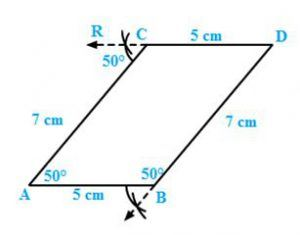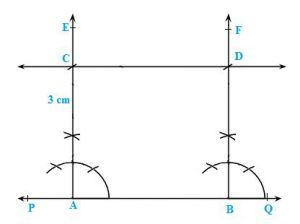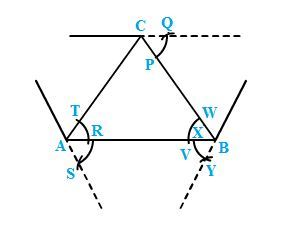Click to Chat

1800-1023-196

+91-120-4616500

CART 0

• 0

MY CART (5)

Use Coupon: CART20 and get 20% off on all online Study Material

ITEM
DETAILS
MRP
DISCOUNT
FINAL PRICE
Total Price: Rs.

There are no items in this cart.
Continue Shopping• Complete JEE Main/Advanced Course and Test Series
• OFFERED PRICE: Rs. 15,900
• View Details

```Constructions Exercise 17.1

Question: 1

Draw an ∠BAC of measure 50° such that AB = 5 cm and AC = 7 cm. Through C draw a line parallel to AB and through B draw a line parallel to AC, intersecting each other at D. Measure BD and CD

Solution:Steps of construction:

Draw angle BAC = 50° such that AB = 5 cm and AC = 7 cm.

Cut an arc through C at an angle of 50°

Draw a straight line passing through C and the arc. This line will be parallel to AB since ∠CAB =∠RCA=50°

Alternate angles are equal; therefore the line is parallel to AB.

Again through B, cut an arc at an angle of 50° and draw a line passing through B and this arc and say this intersects the line drawn parallel to AB at D.

∠SBA =∠BAC = 50°, since they are alternate angles. Therefore BD parallel to AC

Also we can measure BD = 7 cm and CD = 5 cm.

Question: 2

Draw a line PQ.  Draw another line parallel to PQ at a distance of 3 cm from it.

Solution:Steps of construction:

Draw a line PQ.

Take any two points A and B on the line.

Construct ∠PBF = 90° and ∠QAE = 90°

With A as centre and radius 3 cm cut AE at C.

With B as centre and radius 3 cm cut BF at D.

Join CD and produce it on either side to get the required line parallel to AB and at a distance of 5 cm from it.

Question: 3

Take any three non-collinear points A, B, C and draw ∠ABC. Through each vertex of the triangle, draw a line parallel to the opposite side.

Solution:Steps of construction:

Mark three non collinear points A, B and C such that none of them lie on the same line.

Join AB, BC and CA to form triangle ABC.

Parallel line to AC

With A as centre, draw an arc cutting AC and AB at T and U, respectively.

With centre B and the same radius as in the previous step, draw an arc on the opposite side of AB to cut AB at X.

With centre X and radius equal to TU, draw an arc cutting the arc drawn in the previous step at Y.

Join BY and produce in both directions to obtain the line parallel to AC.

Parallel line to AB

With B as centre, draw an arc cutting BC and BA at W and V, respectively.

With centre C and the same radius as in the previous step, draw an arc on the opposite side of BC to cut BC at P.

With centre P and radius equal to WV, draw an arc cutting the arc drawn in the previous step at Q.

Join CQ and produce in both directions to obtain the line parallel to AB.

Parallel line to BC

With B as centre, draw an arc cutting BC and BA at W and V, respectively (already drawn).

With centre A and the same radius as in the previous step, draw an arc on the opposite side of AB to cut AB at R.

With centre R and radius equal to WV, draw an arc cutting the arc drawn in the previous step at S.

Join AS and produce in both directions to obtain the line parallel to BC.

Question: 4

Draw two parallel lines at a distance of 5kms apart.

Solution:Steps of construction:

Draw a line PQ.

Take any two points A and B on the line.

Construct ∠PBF = 90° and ∠QAE = 90°

With A as centre and radius 5 cm cut AE at C.

With B as centre and radius 5 cm cut BF at D.

Join CD and produce it on either side to get the required line parallel to AB and at a distance of 5 cm from it.
```### Course Features

• 728 Video Lectures
• Revision Notes
• Previous Year Papers
• Mind Map
• Study Planner
• NCERT Solutions
• Discussion Forum
• Test paper with Video Solution